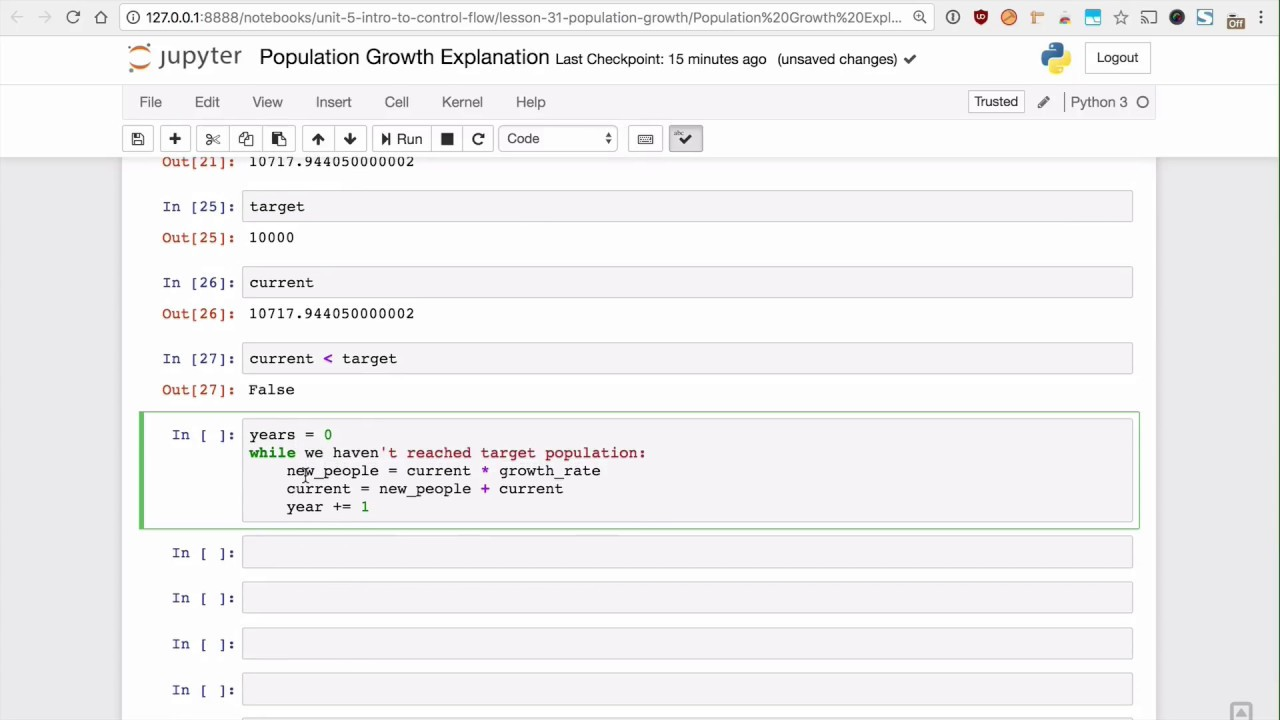# Population growth

Define a function population_growth that receives an initial population,
annual rate of growth, and target population. Use a while loop to calculate
how many years it takes to go over the target population and return it. If
the annual growth rate is not greater than zero, return 'invalid growth rate'.
Do not use math.pow or the x**y operator.

Target = Initial * (1 + rate)^(number of years)

Examples:

>>> population_growth(5000, 0.1, 10000)
8
>>> population_growth(5000, 0.1, 20000)
15
>>> population_growth(5000, 0.1, 40000)
22
>>> population_growth(5000, 0, 40000)
'invalid growth rate'


### Test Cases

test population X2 -

def test_population_X2():
assert population_growth(5000, 0.1, 10000) == 8


test population X4 -

def test_population_X4():
assert population_growth(5000, 0.1, 20000) == 15


test population X8 -

def test_population_X8():
assert population_growth(5000, 0.1, 40000) == 22


def test_bad_growth_rate():
assert population_growth(5000, 0, 40000) == 'invalid growth rate'


Recorded solutionSolution 1

def population_growth(initial_pop, annual_growth_rate, target_pop):
# Initial check, let's make sure it's a valid growth rate
if annual_growth_rate <= 0:
return 'invalid growth rate'

# All good! Let's start the actual algo:

current_pop = initial_pop
num_years_passed = 0
while current_pop <= target_pop:
current_pop *= (1 + annual_growth_rate)
num_years_passed += 1
return num_years_passed

##### Files associated with this lesson:

Population Growth Explanation.ipynb

def population_growth(initial_pop, annual_growth_rate, target_pop): pass